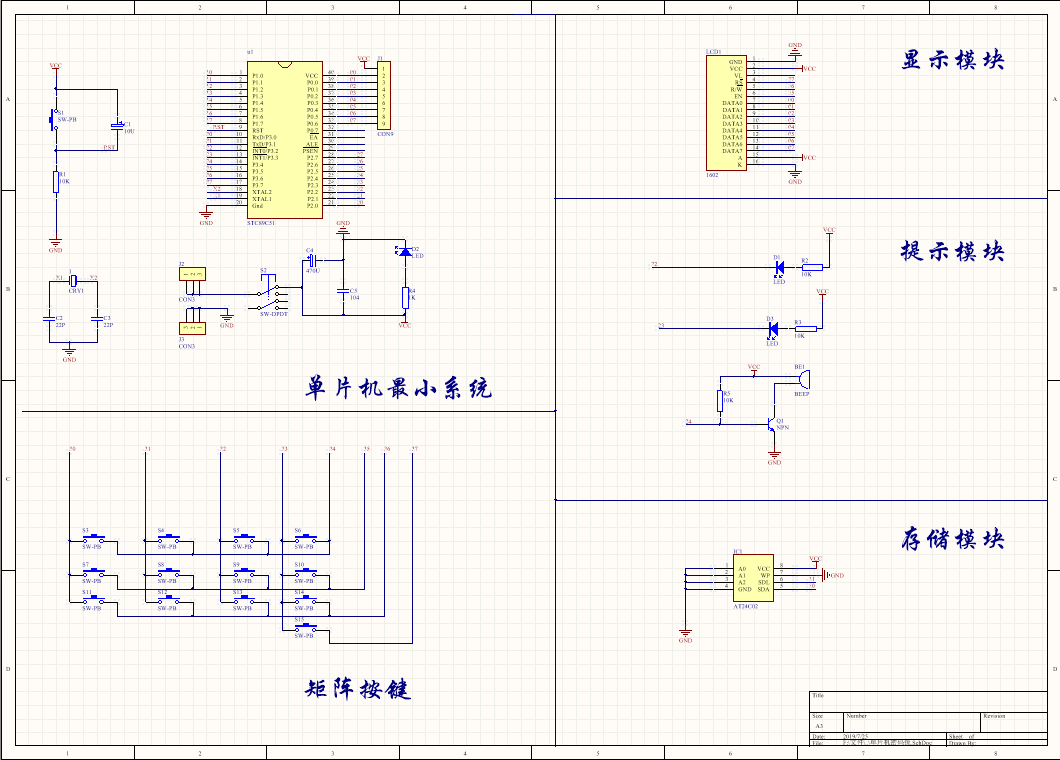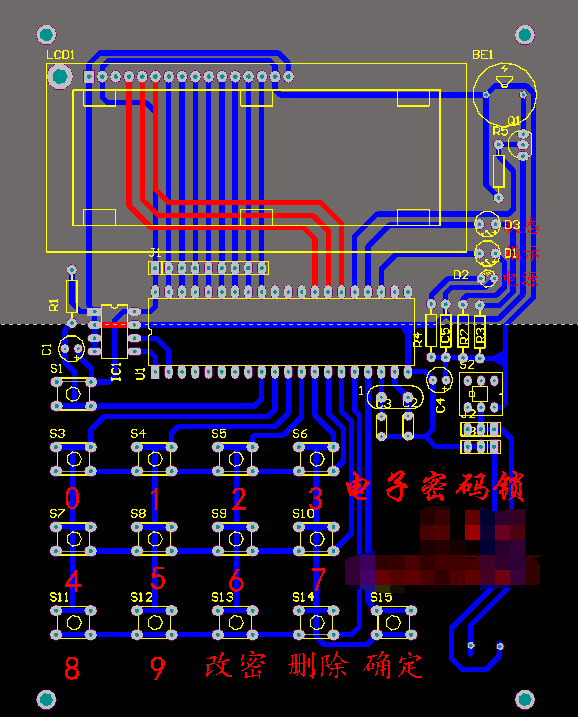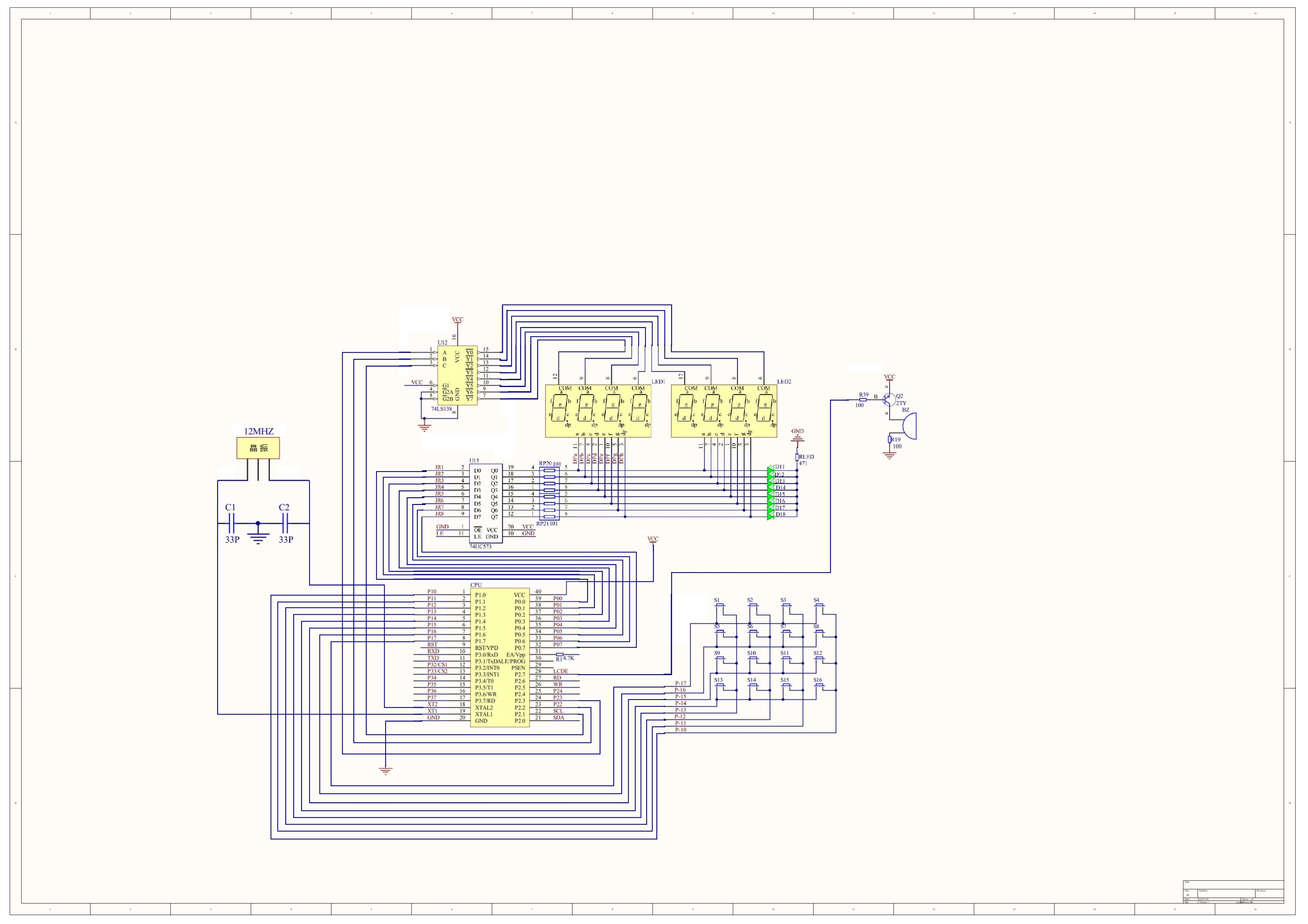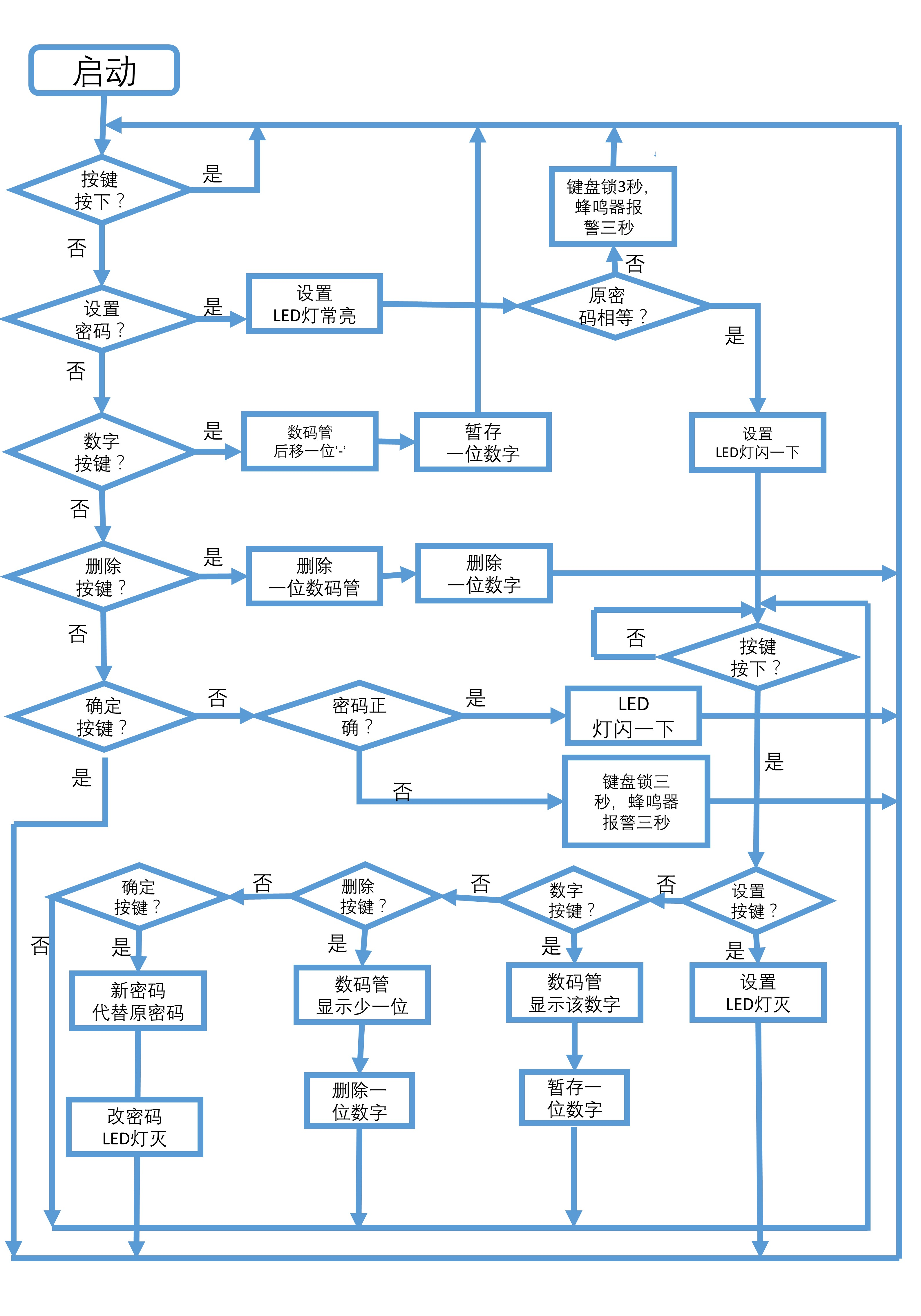2019-07-25 17:12:37 weixin_42107954 阅读数 979
• ###### 单片机控制第一个外设-LED灯-第1季第6部分

本课程是《朱有鹏老师单片机完全学习系列课程》第1季第6个课程，主要讲解LED的工作原理和开发板原理图、实践编程等，通过学习目的是让大家学会给单片机编程控制LED灯，并且为进一步学习其他外设打好基础。

3997 人正在学习 去看看 朱有鹏2011-04-01 22:51:00 missha126 阅读数 2280
• ###### 单片机控制第一个外设-LED灯-第1季第6部分

本课程是《朱有鹏老师单片机完全学习系列课程》第1季第6个课程，主要讲解LED的工作原理和开发板原理图、实践编程等，通过学习目的是让大家学会给单片机编程控制LED灯，并且为进一步学习其他外设打好基础。

3997 人正在学习 去看看 朱有鹏

arm完成较为简单， 功用较为完善， 用单片机控制的密码锁常运用汇编言语编写程序， 显示器少数用数码管。 便于修正和增减功用；同时采用显示清楚， 显示愈加直观， 采用STC89C52单片机设计出一种具有密码设置、报警和防止屡次试探密码功用的电子密码锁， 运用4×4矩阵键盘作为数据输出方式， 单片机通过密码逐个比较， 如果输出的6位密码和设定的密码完全相同， 那么能成功驱动开锁模仿灯将锁打开。 若输出6位密码与设定的密码不相同， 按下BESURE键后， 但是系统不允许有限次地按BACK键， 以免密码被套用， 当3次密码输出都错误， 单片机将驱动蜂鸣器报警。 并且本系统输出密码还有工夫限制， 若在规则的工夫内没有将正确密码输出也会报警。 按下SET键可新设置密码。 单片机将其送给E2PROM， 系统将自动回到程序末尾， 重新读取密码并保管， 　　2．2软件设计　　本系统软件包括主程序模块、密码比较判别模块、修正密码模块、键盘扫描模块、液晶显示模块及定时程序等模块。 系统程C言语序流程如图1所示。 　　2．2．1主程序模块　　主程序主要用于定义全局变量， 给全局变量赋初值， 启动定时器以及从AT24C02中读取密码， 若密码完全正确则开锁；若不正确， 重新输出密码， 当3次都出错则报警。 　　2．2．3密码修正模块　　在密码输出正确情况下，

2019-03-17 11:12:49 huashaoguang 阅读数 2104
• ###### 单片机控制第一个外设-LED灯-第1季第6部分

本课程是《朱有鹏老师单片机完全学习系列课程》第1季第6个课程，主要讲解LED的工作原理和开发板原理图、实践编程等，通过学习目的是让大家学会给单片机编程控制LED灯，并且为进一步学习其他外设打好基础。

3997 人正在学习 去看看 朱有鹏

51单片机——电子密码锁

，下面展示同样高亮的 `代码片`.
#include<reg51.h>
unsigned char zt=1;
unsigned char table;
unsigned char smg[]={0xc0,0xf9,0xa4,0xb0,0x99,0x92,0x82,0xf8,0x80,0x90};
unsigned char i=0;
unsigned char wrong=0;
unsigned char right=0;
unsigned char input=0;
unsigned char temp;
unsigned char j;
unsigned char m=0;
unsigned char k;
unsigned char q;
sbit red=P2^3;
sbit yellow=P2^0;
sbit green=P2^1;
sbit blue=P2^2;
sbit fm=P2^6;
sbit full=P2^5;
sbit igreen=P2^4;
sbit P34=P3^4;
sbit P35=P3^5;
sbit P36=P3^6;
sbit P37=P3^7;
void delay();
void btn(unsigned char a);
void put();
void submit();
void lock();
void check_C1();
void check_C2();
void check_C3();
void check_C4();
void check_button();
void first();
void open();
void close();
void beep();
void ddelay();
void main(){
P2=0x00;
P0=0Xff;
while(1){

``````if(zt==1){
first();
}
if(zt==2){
open();
}
if(zt==3){
close();
}
``````

}
}
void beep(){
unsigned int c;
unsigned int d;
for(d=0;d<200;d++){
for(c=0;c<200;c++){
fm=1;
ddelay();
fm=0;
}
}
}
void ddelay(){
unsigned int b;
for(b=0;b<=30;b++);
}
void delay(){
unsigned int n;
TMOD=0X00;
for(n=0;n<=100;n++){
TH0=0X63;
TL0=0X18;
TR0=1;
while(!TF0);
TF0=0;
}
}
void btn(unsigned char a){
if(i<8&&input1){
table[i++]=a;
blue=1;
P0=smg[a];
delay();
blue=0;
P0=0xff;
}
if(i
8&&input1){
igreen=0;
full=1;
}
}
m=0;
for(k=0;k<8;k++){
m++;
break;
}
}
if(m
8){
right=1;
}else{
full=0;
red=1;
delay();
red=0;
wrong++;
}
if(wrong>3){
red=1;
full=0;
beep();
}
}
void put(){
if(input0){
igreen=1;
input=1;
i=0;
}
}
void submit(){
if(zt
1||zt2){
if(i
8){
for(j=0;j<8;j++)
if(zt2)
i=0;
full=0;
input=0;
}
}
if(zt
3&&i==8){
full=0;
input=0;
i=0;
}

``````if(zt==1&&i==8){
zt=2;
yellow=0;
full=0;
input=0;
i=0;
}
``````

}
void lock(){
if(zt2&&input0){
zt=3;
green=0;
}
}
void check_C1(){
P3=0xff;
P34=0;
temp=P3;
temp=temp&0x0f;
if(temp!=0x0f){
temp=P3;
temp=temp&0x0f;
switch(temp){
case 0x0e:btn(0);break;
case 0x0d:btn(4);break;
case 0x0b:btn(8);break;
case 0x07:break;
}
temp=P3;
temp=temp&0x0f;
while(temp!=0x0f)
{
temp=P3;
temp=temp&0x0f;
}
}
}
void check_C2(){
P3=0xff;
P35=0;
temp=P3;
temp=temp&0x0f;
if(temp!=0x0f){
temp=P3;
temp=temp&0x0f;
switch(temp){
case 0x0e:btn(1);break;
case 0x0d:btn(5);break;
case 0x0b:btn(9);break;
case 0x07:lock();break;
}
temp=P3;
temp=temp&0x0f;
while(temp!=0x0f)
{
temp=P3;
temp=temp&0x0f;
}
}
}
void check_C3(){
P3=0xff;
P36=0;
temp=P3;
temp=temp&0x0f;
if(temp!=0x0f){
temp=P3;
temp=temp&0x0f;
switch(temp){
case 0x0e:btn(2);break;
case 0x0d:btn(6);break;
case 0x0b:break;
case 0x07:put();break;
}
temp=P3;
temp=temp&0x0f;
while(temp!=0x0f)
{
temp=P3;
temp=temp&0x0f;
}
}
}
void check_C4(){
P3=0xff;
P37=0;
temp=P3;
temp=temp&0x0f;
if(temp!=0x0f){
temp=P3;
temp=temp&0x0f;
switch(temp){
case 0x0e:btn(3);break;
case 0x0d:btn(7);break;
case 0x0b:break;
case 0x07:submit();break;
}
temp=P3;
temp=temp&0x0f;
while(temp!=0x0f)
{
temp=P3;
temp=temp&0x0f;
}
}
}
void check_button(){
check_C1();
check_C2();
check_C3();
check_C4();
}

void first(){
yellow=1;
check_button();
while(input1){
check_button();
}
}
void open(){
green=1;
check_button();
while(input
1){
check_button();
}
}
void close(){
yellow=1;
check_button();
while(input1){
check_button();
}
if(right
1){
yellow=0;
red=0;
wrong=0;
right=0;
zt=2;
fm=0;
}
}

2013-10-23 16:35:55 shaozhanyu 阅读数 1513
• ###### 单片机控制第一个外设-LED灯-第1季第6部分

本课程是《朱有鹏老师单片机完全学习系列课程》第1季第6个课程，主要讲解LED的工作原理和开发板原理图、实践编程等，通过学习目的是让大家学会给单片机编程控制LED灯，并且为进一步学习其他外设打好基础。

3997 人正在学习 去看看 朱有鹏
4*4键盘输入，可以更改密码，有24C02存储密码。我用的是6位。也可以多几位，更改相应变量即可。很简单。24C02里面我事先写入了初始密码2 3 4 6 7  0  。调试成功，可以直接用。STC89C51RC单片机 ，万能板焊接
//实验板上24C02已写入数据 {2,3,4,6,7,0}
#include<REG52.H>
#include <intrins.H>
#define uchar unsigned char
#define uint unsigned int
char code SKEY={4,8,11,15,3,7,10,14,2,6,0,13,1,5,9,12};
char code act={0xfe,0xfd,0xfb,0xf7};
char data rd={1,9,8,5,1,1};
char ct=0;
sbit contel=P2^1;
sbit cin=P0^3;
sbit set=P0^4;
sbit wrong=P0^5;
sbit SDA=P2^6;
sbit SCL=P2^7;
bdata char com_data;
sbit mos_bit=com_data^7;
sbit low_bit=com_data^0;
void delay_iic(int n);
uchar rd_24c01(char a);
void wr_24c01(char a,char b);
void wtsec();
/**************************************/
void delay_iic(int n)
{
int i;
for(i=1;i<n;i++){}
}

void delay(unsigned int k)
{
unsigned int data i,j;
for(i=0;i<k;i++)
{
for(j=0;j<121;j++)
{;}
}
}

//*************************************
void start()
{
SDA=1;_nop_();_nop_();_nop_();_nop_();
SCL=1;_nop_();_nop_();_nop_();_nop_();
SDA=0;_nop_();_nop_();_nop_();_nop_();
SCL=0;_nop_();_nop_();_nop_();_nop_();
}
//*****************************************
void stop()
{
SDA=0;_nop_();_nop_();_nop_();_nop_();
SCL=1;_nop_();_nop_();_nop_();_nop_();
SDA=1;_nop_();_nop_();_nop_();_nop_();
}
//***********************
void ack()
{
SCL=1;_nop_();_nop_();_nop_();_nop_();
SCL=0;_nop_();_nop_();_nop_();_nop_();
}
//***********************
void shift8(char a)
{
data uchar i;
com_data=a;
for(i=0;i<8;i++)
{
SDA=mos_bit;
SCL=1;_nop_();_nop_();_nop_();_nop_();_nop_();_nop_();
SCL=0;_nop_();_nop_();_nop_();_nop_();_nop_();_nop_();
com_data=com_data*2;
}
}
//******************
uchar rd_24c01(char a)
{
data uchar i,command;
SDA=1;_nop_();_nop_();_nop_();_nop_();
SCL=0;_nop_();_nop_();_nop_();_nop_();
start();
command=160;
shift8(command);
ack();
shift8(a);
ack();
start();
command=161;
shift8(command);
ack();
SDA=1;_nop_();_nop_();_nop_();_nop_();
for(i=0;i<8;i++)
{
com_data=com_data*2;
SCL=1;_nop_();_nop_();_nop_();_nop_();_nop_();_nop_();
low_bit=SDA;
SCL=0;_nop_();_nop_();_nop_();_nop_();_nop_();_nop_();
}
stop();
return(com_data);
}
//*****************
void wr_24c01(char a,char b)
{
data uchar command;
_nop_();_nop_();_nop_();_nop_();_nop_();
SDA=1;_nop_();_nop_();_nop_();_nop_();
SCL=0;_nop_();_nop_();_nop_();_nop_();
start();
command=160;
shift8(command);
ack();
shift8(a);
ack();
shift8(b);
ack();
stop();
_nop_();_nop_();_nop_();_nop_();_nop_();
}

//键盘扫描
char scan_key(void)
{
char i,j,in,ini=0,inj=0,temp,del;
bit find=0;
P1=0x0f;
temp=P1;
if(temp!=0x0f)        //按键有松开过
for(del=10;del>0;del--);     //键盘去抖延迟
if(temp!=0x0f)
{
for(i=0;i<4;i++)
{
P1=act;
in=P1;
in=in>>4;
in=in|0xf0;
for(j=0;j<4;j++)
{
if(act[j]==in)
{find=1;
inj=j;ini=i;
}
}
}
}
if(find==0)return -1;
return (ini*4+inj);
}
//输入函数
void put_secret()
{
char wrt;
char et,ft;
char wt,x=1;
char *p,*t;
p=rd;
t=wrt;
for(wt=0;wt<6;)
{
et=scan_key();
if(et!=-1)
{
wrt[wt]=SKEY[et];
wt=wt+x;
cin=0;
delay(200);
cin=1;
}
}
ct=0;
for (ft=0;ft<6;)
{
if(wrt==rd)
{ct=ct+x; }

ft=ft+x;
}
if(ct==6)
contel=1;
else
wrong=0;
}

void  wtsec()
{
char t;
char e,f,i=10;
char w,x=1;
char *m,*n;
m=rd;
n=t;
for(w=0;w<6;)
{
e=scan_key();
if(e!=-1)
{
t[w]=SKEY[e];
w=w+x;
cin=0;
delay(200);
cin=1;
}
}
for (f=0;f<6;)
{
wr_24c01(i,t[f]);
delay_iic(250);
rd[f]=rd_24c01(i);
delay_iic(250);
f=f+x;
i=i+x;
}
}
//============================
void main(void)
{
char c,f, key_val,x=1,ii=10;
contel=0;
wrong=1;
cin=1;
set=0;
delay(2000);
for (f=0;f<6;)
{
rd[f]=rd_24c01(ii);
delay_iic(250);
f=f+x;
ii=ii+x;
}
set=1;
delay(1000);
while(1)
{
c=scan_key();
if(c!=-1)
key_val=SKEY[c];
switch(key_val)
{
case 12:cin=0;delay(1000);cin=1;put_secret();break ;
case 13:ct=0;wrong=1;contel=0;cin=1;set=1;break;
case 14:if(ct==6){set=0;wtsec();set=1;} set=1;ct=0;break;
default:break;
}
}
}

2019-02-05 22:09:48 weixin_43237195 阅读数 2406
• ###### 单片机控制第一个外设-LED灯-第1季第6部分

本课程是《朱有鹏老师单片机完全学习系列课程》第1季第6个课程，主要讲解LED的工作原理和开发板原理图、实践编程等，通过学习目的是让大家学会给单片机编程控制LED灯，并且为进一步学习其他外设打好基础。

3997 人正在学习 去看看 朱有鹏

1个蜂鸣器
1个4×4矩阵按键
1个51单片机最小系统
2个LED灯
8位数码管。## 软件设计 程序设计流程图 -``````ORG 0000H
MOV P0,#00H
MOV P2,#0FFH
LJMP MAIN1
ORG 0100H

MAIN1:

MOV 21H,#00H  //所有状态清零
MOV 22H,#00H
MOV 23H,#00H
MOV 24H,#00H
MOV 25H,#00H
MOV 26H,#00H
MOV 27H,#00H
MOV 28H,#00H

MOV 51H,#00H
MOV 52H,#00H
MOV 53H,#00H
MOV 54H,#00H
MOV 55H,#00H
MOV 56H,#00H
MOV 57H,#00H
MOV 58H,#00H

MOV 71H,#00H
MOV 72H,#00H
MOV 73H,#00H
MOV 74H,#00H
MOV 75H,#00H
MOV 76H,#00H
MOV 77H,#00H
MOV 78H,#00H

MOV 33H,#00H
MOV 50H,#00H

MOV 21H,#06H  //设置初始密码
MOV 22H,#03FH
MOV 23H,#066H
MOV 24H,#05BH
MOV 25H,#06FH
MOV 26H,#05BH
MOV 27H,#066H
MOV 28H,#066H

MOV R0,#51H  //两个指针清零
MOV R1,#71H

MAIN:
MOV 50H,#00H

ACALL SAOMIAO
MOV A,50H
CJNE A,#00H,QWER  //判断是否有键盘输入
LJMP QWER3

QWER:
CJNE A,#03H,QWER9  //判断是否要重置密码
JB P2.7,ERERER  //判断当前是否为改密码的状态，二者之间跳转。其中p2.7为重置密码标志位，1：正常状态；0：修改密码状态
MOV 33H,#00H
SETB P2.7

MOV R0,#51H  //状态置零
MOV R1,#71H

MOV 51H,#00H
MOV 52H,#00H
MOV 53H,#00H
MOV 54H,#00H
MOV 55H,#00H
MOV 56H,#00H
MOV 57H,#00H
MOV 58H,#00H

MOV 71H,#00H
MOV 72H,#00H
MOV 73H,#00H
MOV 74H,#00H
MOV 75H,#00H
MOV 76H,#00H
MOV 77H,#00H
MOV 78H,#00H

LJMP QWER3
ERERER:
MOV 33H,#0FFH  //33h中为0ffh则为改密码状态，00h不是改密码状态，此处与上面的p2.7相比有点多余。
CLR P2.7

MOV R0,#51H
MOV R1,#71H
MOV 51H,#00H
MOV 52H,#00H
MOV 53H,#00H
MOV 54H,#00H
MOV 55H,#00H
MOV 56H,#00H
MOV 57H,#00H
MOV 58H,#00H

MOV 71H,#00H
MOV 72H,#00H
MOV 73H,#00H
MOV 74H,#00H
MOV 75H,#00H
MOV 76H,#00H
MOV 77H,#00H
MOV 78H,#00H

LJMP QWER3

QWER9:
CJNE A,#02H,QWER8  //判断是否按了确定键
LCALL PANDUAN//调用判断函数

MOV R0,#51H  //判断结束后，状态清零
MOV R1,#71H

MOV 51H,#00H
MOV 52H,#00H
MOV 53H,#00H
MOV 54H,#00H
MOV 55H,#00H
MOV 56H,#00H
MOV 57H,#00H
MOV 58H,#00H

MOV 71H,#00H
MOV 72H,#00H
MOV 73H,#00H
MOV 74H,#00H
MOV 75H,#00H
MOV 76H,#00H
MOV 77H,#00H
MOV 78H,#00H

LJMP QWER3

QWER8:
CJNE A,#01H,QWER2  //判断是否退格按键按下
CJNE R0,#51H,JIAN   //判断是否超出了八位数码管范围！这个特别重要！（低于0位）
SJMP QWER3
JIAN:
DEC R0  //退格执行语句
DEC R1
MOV @R0,#00H
MOV @R1,#00H
SJMP QWER3
QWER2:
CJNE R0,#59H,JIA  //判断是否超出了八位数码管范围！这个特别重要！（高于8位）
SJMP QWER3
JIA:
MOV @R0,#040H  //按下数字按键则累加
MOV @R1,50H
INC R0
INC R1

QWER3:
SETB P2.4  //八位数码管显示函数，为动态扫描形，p2.2、3、4为138译码器ABC接口  p0为数码管控制位
SETB P2.3
SETB P2.2
MOV P0,51H
ACALL DELAY1  //一定要记得调用延迟函数，不然会出现重影的现象

SETB P2.4
SETB P2.3
CLR P2.2
MOV P0,52H
ACALL DELAY1

SETB P2.4
CLR P2.3
SETB P2.2
MOV P0,53H
ACALL DELAY1

SETB P2.4
CLR P2.3
CLR P2.2
MOV P0,54H
ACALL DELAY1
CLR P2.4
SETB P2.3
SETB P2.2
MOV P0,55H

ACALL DELAY1
CLR P2.4
SETB P2.3
CLR P2.2
MOV P0,56H

ACALL DELAY1
CLR P2.4
CLR P2.3
SETB P2.2
MOV P0,57H
ACALL DELAY1

CLR P2.4
CLR P2.3
CLR P2.2
MOV P0,58H
ACALL DELAY1

LJMP MAIN   //循环回去，相当于C语言里面的while（1）

PANDUAN:  //判断子函数，一位一位判断，不对则调用BEE函数，蜂鸣器报警

MOV A,71H
CJNE A,21H,BEE
MOV A,72H
CJNE A,22H,BEE
MOV A,73H
CJNE A,23H,BEE
MOV A,74H
CJNE A,24H,BEE
MOV A,75H
CJNE A,25H,BEE
MOV A,76H
CJNE A,26H,BEE
MOV A,77H
CJNE A,27H,BEE
MOV A,78H
CJNE A,28H,BEE
MOV A,33H

CJNE A,#0FFH,OVER  //如果都对，判断是否当前为改密码时验证原密码的状态，如果是的，则调用改密码函数，如果不是则正常打开锁（改密码的重点）
MOV 33H,#00H   //清除33h单元标志位
SETB P2.7
LCALL DELAY
LCALL DELAY  //当然如果是改密码状态则p2.7灭一下后又亮，提示用户可以输入新密码了！
LCALL DELAY
CLR P2.7
LCALL SHEZHI	  //调用设置新密码函数

OVER:  //正常开锁状态
CLR P2.0
LCALL DELAY
LCALL DELAY
LCALL DELAY
SETB P2.0
MOV A,33H  //清除33h单元标志位，这里为出现bug所设置的，防止其他操作
RET

BEE:  //蜂鸣器报警函数，无源蜂鸣器，需要高低电平来回变
MOV 33H,#00H
SETB P2.7
MOV R5,#20
MOV R7,#0FFH
HAHAHA:
CPL P1.5
LCALL DELAYBEE
DJNZ R7,HAHAHA
MOV R7,#0FFH
DJNZ R5,HAHAHA
RET

SHEZHI:  //设置新密码子函数（最难点）
MOV R0,#51H
MOV R1,#71H	  //当开始清零
MOV 51H,#00H
MOV 52H,#00H
MOV 53H,#00H
MOV 54H,#00H
MOV 55H,#00H
MOV 56H,#00H
MOV 57H,#00H
MOV 58H,#00H
MOV 71H,#00H
MOV 72H,#00H
MOV 73H,#00H
MOV 74H,#00H
MOV 75H,#00H
MOV 76H,#00H
MOV 77H,#00H
MOV 78H,#00H

SHEZHIK:
MOV 50H,#00H  //和上面的正常模式其实很相似，只是我不会嵌套上面的函数，于是重新搞了一个新的函数，没有注释的地方和上面一样
LCALL SAOMIAO
MOV A,50H
CJNE A,#00H,SHEZHI1
LJMP SHEZHI5

SHEZHI1:
CJNE A,#03H,SHEZHI22  //防止用户花里胡哨操作，在改密码的时候，又突然返回了。所以全部清零，原密码不动
SETB P2.7
MOV 33H,0FFH
MOV 51H,#00H
MOV 52H,#00H
MOV 53H,#00H
MOV 54H,#00H
MOV 55H,#00H
MOV 56H,#00H
MOV 57H,#00H
MOV 58H,#00H
MOV R0,#51H
MOV 33H,#00H  //清除33h
LJMP QWER3

SHEZHI22:
CJNE A,#01H,SHEZHI2  //是否退格
CJNE R0,#51H,JIAN1
SJMP SHEZHI5
JIAN1:
DEC R0
MOV @R0,#00H
SJMP SHEZHI5

SHEZHI2:
CJNE A,#02H,SHEZHI3  //重点！判断是否输入了确定键，如果是的，把新密码来替换原密码
MOV 21H,51H
MOV 22H,52H
MOV 23H,53H
MOV 24H,54H	  // 新密码来替换原密码
MOV 25H,55H
MOV 26H,56H
MOV 27H,57H
MOV 28H,58H

MOV 51H,#00H
MOV 52H,#00H
MOV 53H,#00H
MOV 54H,#00H  //清零，防止bug
MOV 55H,#00H
MOV 56H,#00H
MOV 57H,#00H
MOV 58H,#00H
MOV R0,#51H
SETB P2.7	  //清除p2.7
LJMP QWER3

SHEZHI3:
CJNE R0,#59H,JIA1
SJMP  SHEZHI5
JIA1:
MOV @R0,50H
INC R0

SHEZHI5:  //数码管显示函数
SETB P2.4
SETB P2.3
SETB P2.2
MOV P0,51H
ACALL DELAY1

SETB P2.4
SETB P2.3
CLR P2.2
MOV P0,52H
ACALL DELAY1

SETB P2.4
CLR P2.3
SETB P2.2
MOV P0,53H
ACALL DELAY1

SETB P2.4
CLR P2.3
CLR P2.2
MOV P0,54H
ACALL DELAY1

CLR P2.4
SETB P2.3
SETB P2.2
MOV P0,55H
ACALL DELAY1

CLR P2.4
SETB P2.3
CLR P2.2
MOV P0,56H
ACALL DELAY1

CLR P2.4
CLR P2.3
SETB P2.2
MOV P0,57H
ACALL DELAY1

CLR P2.4
CLR P2.3
CLR P2.2
MOV P0,58H
ACALL DELAY1

LJMP SHEZHIK  //注意此时不可以跳转到主函数，因为新密码还没有输出完成，所以跳转到上面的设置新密码的函数，来接受下一位密码

SAOMIAO:  //扫描矩阵键盘函数

CLR P2.4
CLR P2.3
CLR P2.2
MOV P0,#00H  //这个是为了方式bug 方式键盘输入到了第八位，继续按键的话，第八位数码管会变得很亮设置的

MOV P1,#0F0H
JNB P1.4,Y0
JNB P1.5,Y1
JNB P1.6,Y2
JNB P1.7,Y3
RET
Y0:
MOV P1,#0FH
MOV 30H,#00H
JNB P1.0,X0
JNB P1.1,X1
JNB P1.2,X2
JNB P1.3,X3
Y1:  //矩阵键盘原理，先扫描列再扫描行，得到的结果进入计算函数来计算对应的按键值（注意51单片机如果高电平引脚与低电平引脚短接，则低电平引脚变为高，高电平引脚不变，所以检测的时候检测1，不检测0！！！）
MOV P1,#0FH
MOV 30H,#01H
JNB P1.0,X0
JNB P1.1,X1
JNB P1.2,X2
JNB P1.3,X3
Y2:
MOV P1,#0FH
MOV 30H,#02H
JNB P1.0,X0  ·//数据暂存到30h单元
JNB P1.1,X1
JNB P1.2,X2
JNB P1.3,X3
Y3:
MOV P1,#0FH
MOV 30H,#03H
JNB P1.0,X0
JNB P1.1,X1
JNB P1.2,X2
JNB P1.3,X3
X0:
MOV 31H,#00H
ACALL DELAY
MOV P1,#0F0H
LJMP JISUAN
X1:
MOV 31H,#01H
ACALL DELAY
MOV P1,#0F0H
LJMP JISUAN
X2:
MOV 31H,#02H
ACALL DELAY
MOV P1,#0F0H
LJMP JISUAN
X3:
MOV 31H,#03H
ACALL DELAY
MOV P1,#0F0H
LJMP JISUAN

JISUAN:  //开始计算，分别把值给50h，这个值是指数码管显示的数值，这个一定要区别
G0:MOV A,30H
CJNE A,#00H,A0  //其中退格是#01h，确定是#02h，设置密码是#03h
MOV A,31H
CJNE A,#00H,G1
MOV 50H,#02H
G1:	CJNE A,#01H,G2
MOV 50H,#03FH
MOV 70H,#040H
G2:	CJNE A,#02H,A0
MOV 50H,#01H
A0:MOV A,30H
CJNE A,#01H,B0
MOV A,31H
CJNE A,#00H,A1
MOV 50H,#04FH
A1:	CJNE A,#01H,A2
MOV 50H,#05BH
A2:	CJNE A,#02H,B0
MOV 50H,#06H
B0:MOV A,30H
CJNE A,#02H,C0
MOV A,31H
CJNE A,#00H,B1
MOV 50H,#07DH
B1:	CJNE A,#01H,B2
MOV 50H,#06DH
B2:	CJNE A,#02H,C0
MOV 50H, #66H
C0:MOV A,30H
CJNE A,#03H,D0
MOV A,31H
CJNE A,#00H,C1
MOV 50H,#06FH
C1:	CJNE A,#01H,C2
MOV 50H,#07FH
C2:	CJNE A,#02H,C3
MOV 50H,#07H
C3:	CJNE A,#03H,D0
MOV 50H,#03H
D0:RET

DELAY1: MOV R4,#0FFH	  //延迟函数
DJNZ R4,\$
RET

DELAYBEE:MOV R4,#50
``````